# Coordinates of midpoint

If the midpoint of the segment is (6,3) and the other end is (8,4) what are the coordinate of the other end?

x =  4
y =  2

### Step-by-step explanation: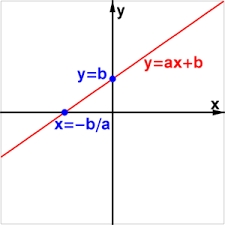Did you find an error or inaccuracy? Feel free to write us. Thank you!Tips to related online calculators
Line slope calculator is helpful for basic calculations in analytic geometry. The coordinates of two points in the plane calculate slope, normal and parametric line equation(s), slope, directional angle, direction vector, the length of the segment, intersections of the coordinate axes, etc.
Looking for a statistical calculator?
Do you have a linear equation or system of equations and looking for its solution? Or do you have a quadratic equation?

## Related math problems and questions:

• Midpoint 4If the midpoint of a segment is (6,3) and the other end point is (8,-4) what are thw coordinate of the other end?
• Midpoint of segmentPoint A has coordinates [4; -11] and the midpoint of the segment AB is the point [17; -7]. What are the coordinates of point B?
• Points OPQ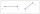Point P is on line segment OQ. Given OP = 6, OQ = 4x - 3, and PQ = 3x, find the numerical length of OQ.
• Cone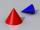If the segment of the line y = -3x +4, that lies in quadrant I, is rotated about the y-axis, a cone is formed. What is the volume of the cone?
• Find the 3Find the distance and midpoint between A(1,2) and B(5,5).
• Line segmentFor the line segment whose endpoints are L[-1, 13] and M[18, 2], find the x and y value for the point located 4 over 7 the distance from L to M.
• Three points 4The line passed through three points - see table: x y -6 4 -4 3 -2 2 Write line equation in y=mx+b form
• Midpoint 6For line segment length is given: FM=8a+1, FG=42. Point M is the midpoint of FG. Find unknown a.
• The coordinates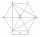The coordinates (5, 2) and (-6, 2) are vertices of a hexagon. Explain how to find the length of the segment formed by these endpoints. How long is the segment?
• Coordinates of the intersection of the diagonals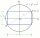In the rectangular coordinate system, a rectangle ABCD is drawn. The vertices of the rectangle are determined by these coordinates A = (2.2) B = (8.2) C = (8.6) D = (2.6) Find the coordinates of the intersection of the diagonals of the ABCD rectangle
• LineStraight-line passing through points A [-3; 22] and B [33; -2]. Determine the total number of points of the line in which both coordinates are positive integers.
• Points on line segmentPoints P & Q belong to segment AB. If AB=a, AP = 2PQ = 2QB, find the distance: between point A and the midpoint of the segment QB.
• Coordinates hexagon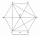The regular hexagon ABCDEF is given. Point A has coordinates [1; 3], and point D has coordinates [4; 7]. Calculate the sum of the coordinates of the center of its described circle.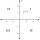Which point is located in Quadrant IV? A coordinate plane. A(-8, 6) B(-8, -6) C(8, -6) D(8, 6)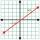What is the slope of the perpendicular bisector of line segment AB if A[9,9] and B[9,-2]?Consider the following ordered pairs that represent a relation. {(–4, –7), (0, 6), (5, –3), (5, 2)} What can be concluded of the domain and range for this relation?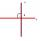Determine the slope of the line perpendicular to the line p: y = -x +4.